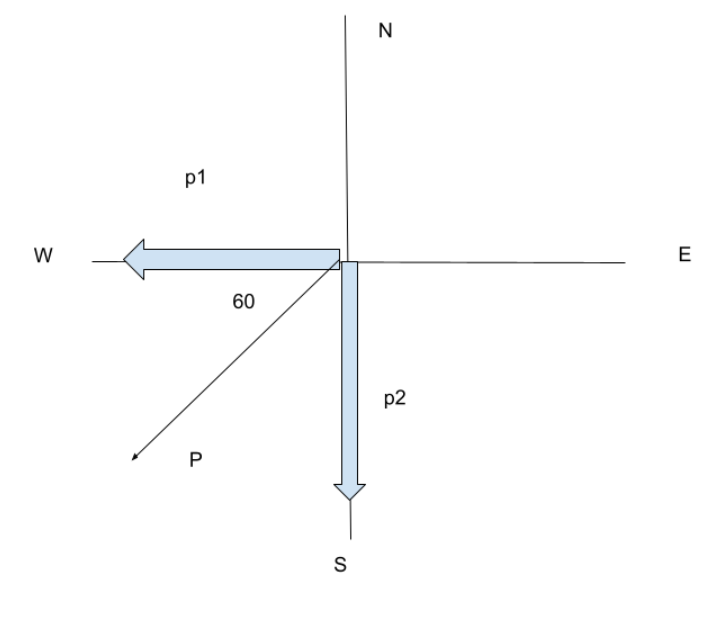×
Get Full Access to Probability And Statistics For Engineers And The Scientists - 9 Edition - Chapter 3 - Problem 51e
Get Full Access to Probability And Statistics For Engineers And The Scientists - 9 Edition - Chapter 3 - Problem 51e

×

# Refer to the previous exercise. a. What is the expectedISBN: 9780321629111 32

## Solution for problem 51E Chapter 3

Probability and Statistics for Engineers and the Scientists | 9th Edition

• Textbook Solutions
• 2901 Step-by-step solutions solved by professors and subject experts
• Get 24/7 help from StudySoup virtual teaching assistantsProbability and Statistics for Engineers and the Scientists | 9th Edition

4 5 1 350 Reviews
20
3
Problem 51E

Three cards are drawn without replacement from the 12 face cards (jacks, queens, and kings) of an ordinary deck of 52 playing cards. Let $$X$$ be the number of kings selected and $$Y$$ the number of jacks. Find

(a) the joint probability distribution of $$X$$ and $$Y$$;

(b) $$P[(X, Y) \in A]$$, where $$A$$ is the region given by $$\{(x, y) \mid x+y \geq 2\}$$

Step-by-Step Solution:

Step 1 of 2

The total momentum is, $$P=7200 \mathrm{~kg} \mathrm{~m} / \mathrm{s}$$ towards $$60^{0}$$ west of north.

The two masses are, $$m_{1}=1500 \mathrm{~kg}$$ and $$m_{2}=2000 \mathrm{~kg}$$.Step 2 of 2

##### ISBN: 9780321629111

The full step-by-step solution to problem: 51E from chapter: 3 was answered by , our top Statistics solution expert on 05/06/17, 06:21PM. This full solution covers the following key subjects: among, calls, involve, fax, message. This expansive textbook survival guide covers 18 chapters, and 1582 solutions. Probability and Statistics for Engineers and the Scientists was written by and is associated to the ISBN: 9780321629111. This textbook survival guide was created for the textbook: Probability and Statistics for Engineers and the Scientists, edition: 9. Since the solution to 51E from 3 chapter was answered, more than 1281 students have viewed the full step-by-step answer. The answer to “?Three cards are drawn without replacement from the 12 face cards (jacks, queens, and kings) of an ordinary deck of 52 playing cards. Let $$X$$ be the number of kings selected and $$Y$$ the number of jacks. Find(a) the joint probability distribution of $$X$$ and $$Y$$;(b) $$P[(X, Y) \in A]$$, where $$A$$ is the region given by $$\{(x, y) \mid x+y \geq 2\}$$” is broken down into a number of easy to follow steps, and 63 words.

## Discover and learn what students are asking

Statistics: Informed Decisions Using Data : Comparing Three or More Means (One-Way Analysis of Variance)
?The variability within each treatment group, which is a weighted average of the sample variances from each treatment where the weights are based on th

Statistics: Informed Decisions Using Data : Inference about the Difference between Two Medians: Dependent Samples
?Car Rentals The following data represent the weekday rental rate for a compact car charged by two car-rental companies, Avis and Hertz, in 11 location

Unlock Textbook Solution